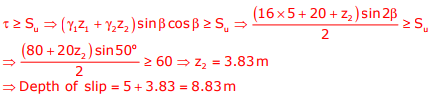# Geotechnical Engineering - Online Test

Q1. A 6 m high retaining wall having a smooth vertical back face retains a layered horizontal backfill. Top 3 m thick layer of the backfill is sand having an angle of internal friction, φ = 30° while the bottom layer is 3 m thick clay with cohesion, c = 20 kPa. Assume unit weight for both sand and clay as 18 kN/m3. The totalactive earth pressure per unit length of the wall (in kN/m) is:
Explaination / Solution:
No Explaination.

Q2. As per Indian Standard Soil Classification System (IS: 1498 - 1970), an expression for A-line is
Explaination / Solution:
No Explaination.

Q3. The clay mineral primarily governing the swelling behavior of Black Cotton soil is
Explaination / Solution:
No Explaination.

Q4. Group I enlists in-situ field tests carried out for soil exploration, while Group II provides a list of parameters for sub-soil strength characterization. Match the type of tests with the characterization parameters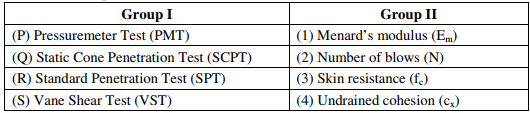Explaination / Solution:
No Explaination.

Q5. Following statement are made on compacted soil, where DS stands for soil compaction on Dry Side of OMC and WS stands for soil compacted on Wet Side of OMC. Identify incorrect statement
Explaination / Solution:
No Explaination.

Q6. For a saturated sand deposit, the void ratio and the specific gravity of solids are 0.70 and 2.67, respectively. The critical (upward) hydraulic gradient for the deposit would be
Explaination / Solution:
No Explaination.

Q7. For a sample of dry, cohesionless soil with friction angle,Ø  the failure plane will be inclined to the major principal plane by an angle equal to
Explaination / Solution:
No Explaination.

Q8. A soil is composed of solid spherical grains of identical specific gravity and diameter between 0.075mm and 0.0075mm. If the terminal velocity of the largest particle falling through water without flocculation is 0.5mm/s, that for the smallest particle would be
Explaination / Solution:
No Explaination.

Q9. Two soil profile are used as backfill behind a retaining wall as shown in the figure, where γt is total unit weight and c’ & φ' ‘ are effective shear parameters. Find the resultant active earth pressure in kN/m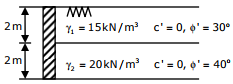Explaination / Solution:Q10. The soil profile above the rock surface at 25o infinite slope is shown in figure where Su is undrain shear stress and γt is total unit weight. The slip will occur at a depth of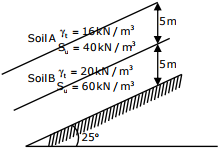Explaination / Solution: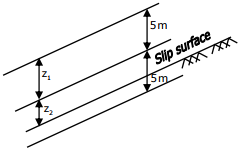The slip will occur when shear stress is greater than or equal to shear strength